#Linear SystemsMost of real life problems involve nonlinear systems (the predator-prey model is one such example). The nonlinear systems are very hard to solve explicitly, but qualitative and numerical techniques may help shed some information on the behavior of the solutions. But there are examples which are modeled by linear systems (the spring-mass model is one of them). Recall that a linear system of differential equations is given as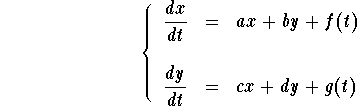Example: The Harmonic Oscillator
This is a model for the motion of mass attached to a spring. Let x(t) be the displacement (which is the position of the mass from the equilibrium position or the rest position). Newton's Law of mechanics gives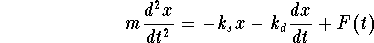,

where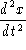is the acceleration,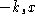is the restoring force provided by the spring,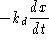is the damping force, and F(t) is an external force acting on the mass (such as an electrical field or a magnetic field for example). This is a second order differential equation. It may be translated to a system of first order differential system as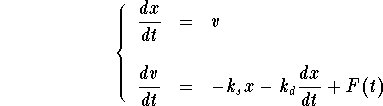where v is the velocity of the mass at time t. Clearly we have a linear system.

Definitions:

If f(t)=g(t) = 0, then the linear system is called homogeneous and reduces to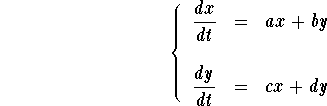Otherwise, it is called nonhomogeneous.

a, b, c, d are called the coefficients of the system. If all are constants then the system is said to be linear with constant coefficients.

Clearly, we may use our previous knowledge about systems for the linear ones. For example, we may associate the direction field to the linear system as well.

Example: Draw the direction field of the system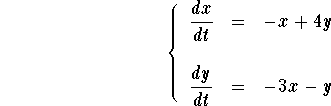as well as some solutions.

Answer. Below is the direction field of the system. Red vectors symbolize "long" vectors, light blue vectors symbolize slow motion. The second picture also shows some typical solutions. The initial value conditions are indicated by dots.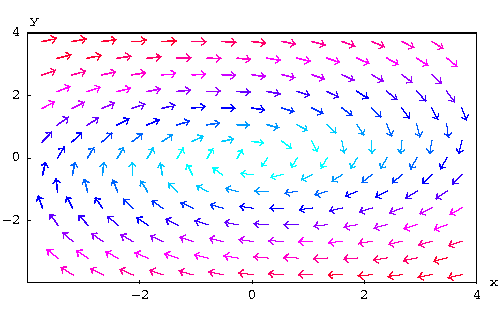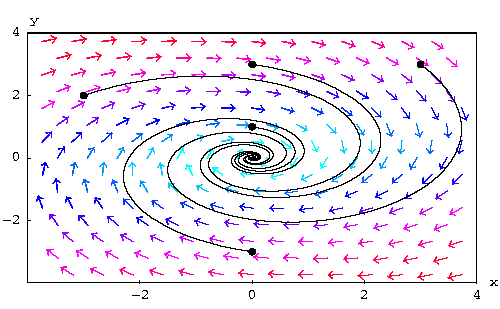[Differential Equations] [First Order D.E.]
[Geometry] [Algebra] [Trigonometry ]
[Calculus] [Complex Variables] [Matrix Algebra]S.O.S MATHematics home page

Do you need more help? Please post your question on our S.O.S. Mathematics CyberBoard.Author: Mohamed Amine Khamsi